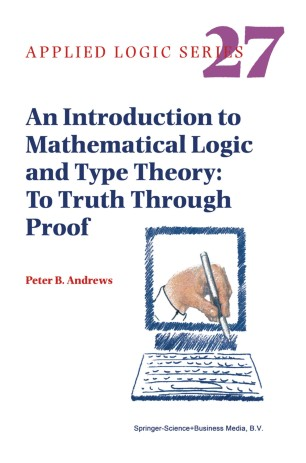# An Introduction to Mathematical Logic and Type Theory: To Truth Through Proof

• Peter B. AndrewsTextbook

Part of the Applied Logic Series book series (APLS, volume 27)

1. Front Matter
Pages i-xviii
2. Peter B. Andrews
Pages 1-3
3. Peter B. Andrews
Pages 5-72
4. Peter B. Andrews
Pages 73-150
5. Peter B. Andrews
Pages 151-188
6. Peter B. Andrews
Pages 189-200
7. Peter B. Andrews
Pages 201-256
8. Peter B. Andrews
Pages 257-299
9. Peter B. Andrews
Pages 301-338
10. Back Matter
Pages 339-390

### Introduction

This introduction to mathematical logic starts with propositional calculus and first-order logic. Topics covered include syntax, semantics, soundness, completeness, independence, normal forms, vertical paths through negation normal formulas, compactness, Smullyan's Unifying Principle, natural deduction, cut-elimination, semantic tableaux, Skolemization, Herbrand's Theorem, unification, duality, interpolation, and definability.

The last three chapters of the book provide an introduction to type theory (higher-order logic). It is shown how various mathematical concepts can be formalized in this very expressive formal language. This expressive notation facilitates proofs of the classical incompleteness and undecidability theorems which are very elegant and easy to understand. The discussion of semantics makes clear the important distinction between standard and nonstandard models which is so important in understanding puzzling phenomena such as the incompleteness theorems and Skolem's Paradox about countable models of set theory.

Some of the numerous exercises require giving formal proofs. A computer program called ETPS which is available from the web facilitates doing and checking such exercises.

Audience: This volume will be of interest to mathematicians, computer scientists, and philosophers in universities, as well as to computer scientists in industry who wish to use higher-order logic for hardware and software specification and verification.

### Keywords

Syntax formal language formal proof logic mathematical logic predicate logic proof propositional calculus semantics set theory type theory verification

#### Authors and affiliations

• Peter B. Andrews
• 1
1. 1.Department of Mathematical SciencesCarnegie Mellon UniversityPittsburghUSA

### Bibliographic information

• DOI https://doi.org/10.1007/978-94-015-9934-4